Next: Traps for the unwary Up: Converting Al and Be Previous: Estimating the number of

# Modifications for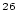Al

This procedure is essentially the same forAl. The only major difference in the chemical processing is that the quartz sample typically contains a relatively large amount of total Al, so it is not necessary to add Al carrier. Thus, the total number of atoms of stable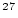Al in the sample, here denoted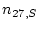, replaces the number of atoms of the stable nuclide added as carrier. This quantity is: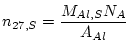(15)

where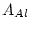is the molar weight of Al (26.982 g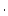mol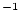) and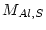is the mass of Al in the sample (g). The mass of Al in the sample is usually determined by ICP-OES or AA analysis of an aliquot taken immediately after the sample has been dissolved. TheAl concentration in quartz,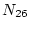, is then: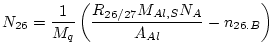(16)

where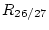is the measuredAl/Al ratio. The uncertainty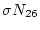is: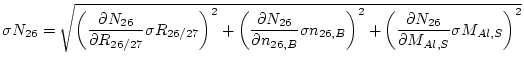(17)

where: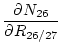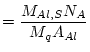(18)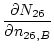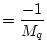(19)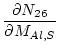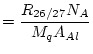(20)

One determines the process blank forAl measurements in the same way as for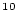Be, as described above.Al is a much less common nuclide in the environment, so generallyAl blanks are less important thanBe blanks.Next: Traps for the unwary Up: Converting Al and Be Previous: Estimating the number of
2006-05-08